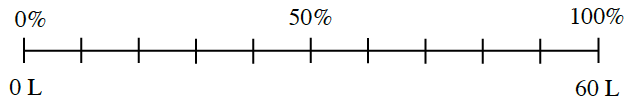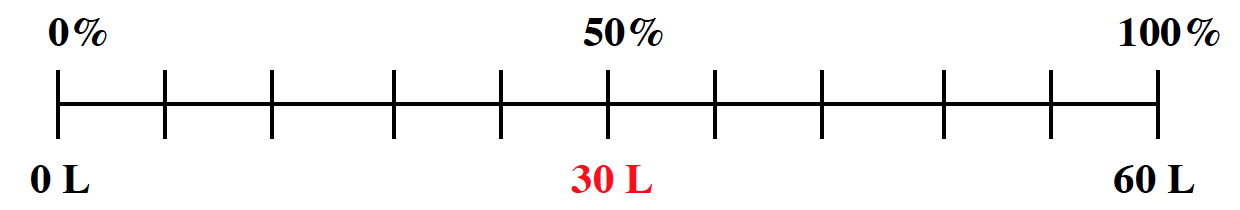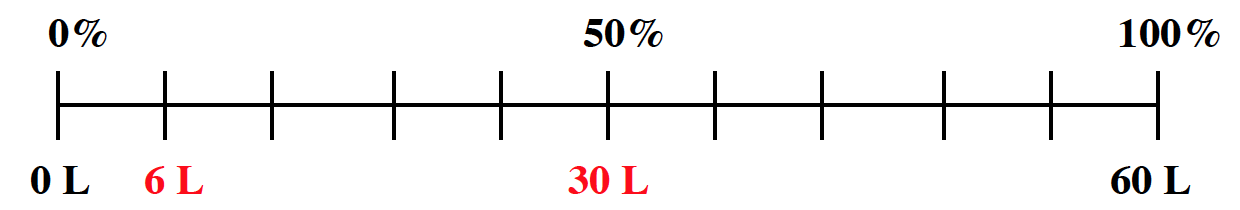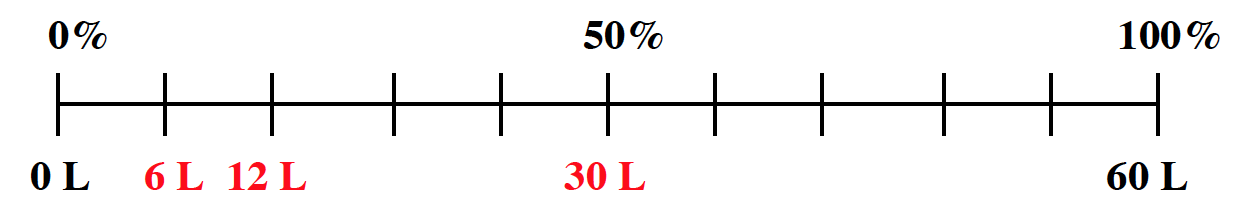### Home > CC1MN > Chapter 3 > Lesson 3.1.3 > Problem3-47

3-47.

Maurice's gas tank can hold $60$ liters of gas. On your paper, copy and label the percent ruler below. Then use it to find how many liters are in the tank when it is:1. $50\%$ full

1. $\frac { 7 } { 10 }$ full

1. $\frac{2}{5}$ full

$50\%$ is equal to one half. What is one half of $60$ liters?

30 L Added to the percent ruler is 30 liters under 50%.

If you divide $60$ liters into $10$ parts, there will be $6$ liters each. How many liters would there be if you had $7$ of these groups? Added to the percent ruler: 6 liters under 10%.

This problem is similar to part (b). When you divide $60$ liters into $5$ parts, each part has $12$ liters. Added to the percent ruler: 12 liters under 20%